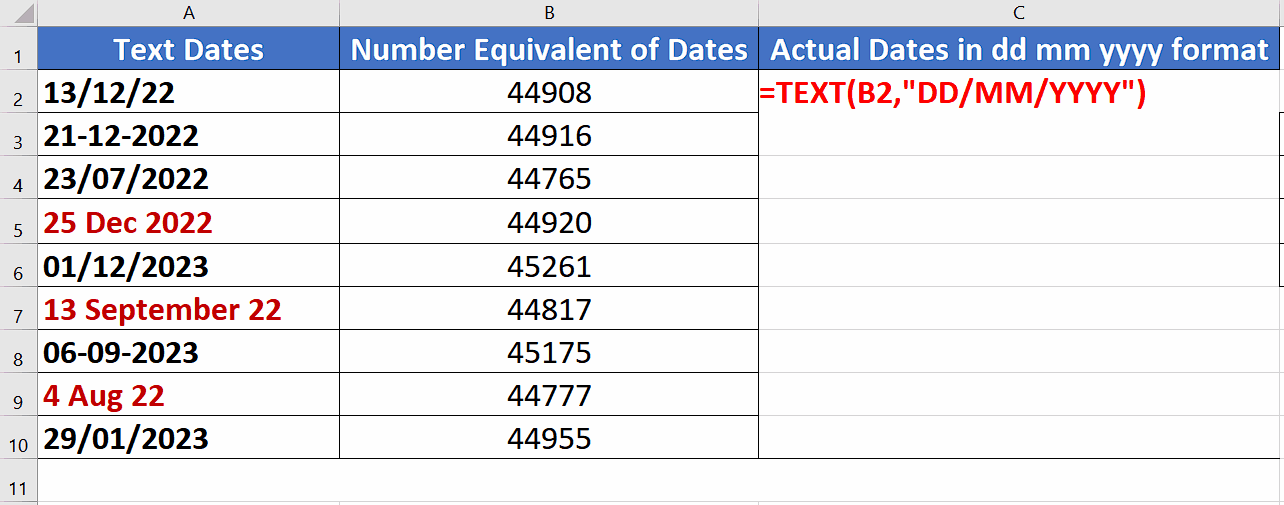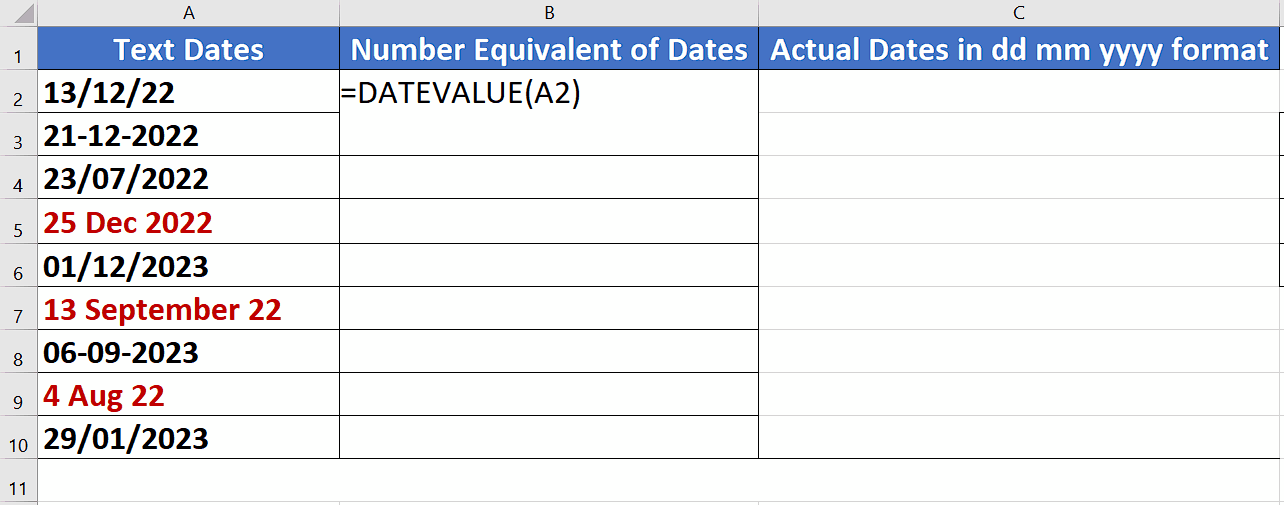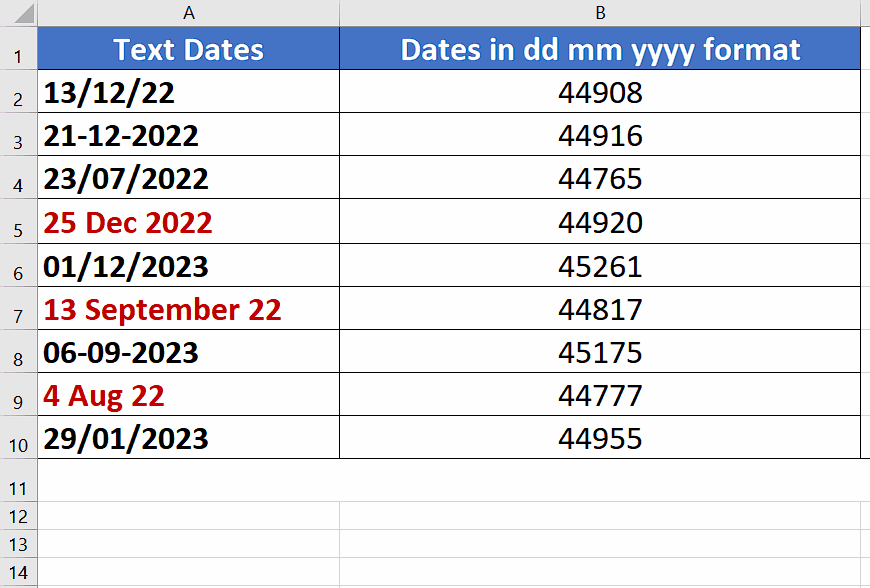# How to convert text to date format dd/mm/yyyy in Excel

Sometimes dates are entered as text by adding an apostrophe ‘ sign before the date and that converts the date into a text date just like our current dataset shown above.

We can see in our data that we have all type of formats of dates in our data. However, all data is recognized as Text by Excel rather than the dates. In this tutorial we’ll learn how to convert these different formats of text dates into actual dates recognized by Excel in the conventional dd/mm/yyyy format. Excel has a very simple built-in function called DATEVALUE(date_text) which accepts the date string i.e., a date in text format and returns an equivalent number. We can then convert that number to a proper date by passing that value to TEXT function which will convert this number into dd/mm/yyyy format.

Let’s follow the steps mentioned below to do it.In Microsoft Excel, a date is a piece of information that represents a particular day. Dates are typically entered into a cell in a specific format e.g., “month/day/year” and can be used in calculations, such as figuring out the number of days between two dates or the future value of an investment. Excel also has a number of built-in functions and formatting options that make working with dates easy and efficient.

### Step 1 – Convert the text dates to equivalent numbers– We’ll first convert the text dates into their equivalent numbers by using the formula =DATEVALUE(A2), since cell A2 has the text date so we used this cell. You can change this as per your dataset.

– This will return us the number equivalent of the text date in A2 as shown above. However, for this to work properly, all dates must be in a valid date format as shown in our dataset.

### Step 2 – Convert all the numbers to dd/mm/yyyy format– We’ll now use the TEXT function to convert the numbers to our required format. We’ll use the formula =TEXT(B2,”dd/mm/yyyy”) to convert the numbers to dates in our required format as shown above. So, write this formula in cell C2 and press enter to get the result.

Double click the fill handle to implement the formula to the whole data range as shown above.

### Alternative of Step 2 – Convert all the numbers to dd/mm/yyyy format– If you don’t want to use the formula to convert the numbers to dd/mm/yyyy format then select all numbers and press CTRL+1.

– Choose Date in the Category options.

– Choose your Locale to be the United Kingdom to show the dates in dd/mm/yyyy format and then choose the same format from Type: field as well as shown above.

– This will convert the numbers to the equivalent dates in the same column and we don’t have to use the new column to change the format of numbers to dates.